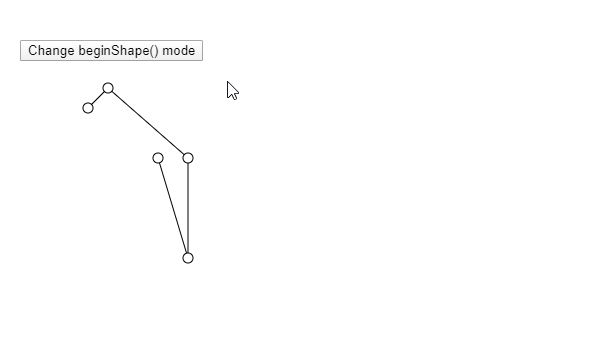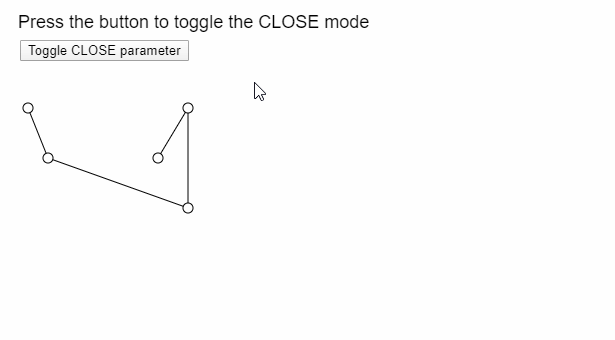# p5.js | endShape() Function

• Last Updated : 27 May, 2020

The endShape() function in p5.js is used after the beginShape() function to finish the drawing of a shape. When this function is called, all the image data defined after the preceding beginShape() function is written to the image buffer to be used as a shape.

There is an optional mode parameter that can be defined to use the “CLOSE” mode. This mode helps to close the shape before ending it.

Syntax:

`endShape( [mode] )`

Parameters: This function accepts one parameter as mentioned above and described below:

• mode: It is a constant that can be used to change the mode of the function. It can have one value CLOSE. This is used to close the shape, that is connect the beginning of the shape to the end. It is an optional parameter.

The examples below illustrates the endShape() function in p5.js:

Example 1:

 `let currMode;`` ` `function` `setup() {``  ``createCanvas(600, 300);``  ``textSize(18);`` ` `  ``let shapeModes = [``    ``LINES,``    ``TRIANGLES,``    ``TRIANGLE_FAN,``    ``TRIANGLE_STRIP,``    ``QUADS``  ``];``  ``let index = 0;``  ``currMode = shapeModes[index];`` ` `  ``let closeBtn = createButton(``"Change beginShape() mode"``);``  ``closeBtn.position(20, 40);``  ``closeBtn.mouseClicked(() => {``    ``if` `(index < shapeModes.length) index++;``    ``else` `index = 0;``    ``currMode = shapeModes[index];``  ``});``}`` ` `function` `draw() {``  ``clear();`` ` `  ``// Starting the shape using beginShape()``  ``beginShape(currMode);``  ``vertex(80, 100);``  ``vertex(100, 80);``  ``vertex(180, 150);``  ``vertex(180, 250);``  ``vertex(150, 150);`` ` `  ``// Ending the shape using endShape()``  ``endShape();`` ` `  ``// Points``  ``circle(80, 100, 10);``  ``circle(100, 80, 10);``  ``circle(180, 150, 10);``  ``circle(180, 250, 10);``  ``circle(150, 150, 10);``}`

Output:Example 2:

 `let isUsingClose = ``false``;`` ` `function` `setup() {``  ``createCanvas(400, 300);``  ``textSize(18);`` ` `  ``let closeBtn = createButton(``"Toggle CLOSE parameter"``);``  ``closeBtn.position(20, 40);``  ``closeBtn.mouseClicked(() => (isUsingClose = !isUsingClose));``}`` ` `function` `draw() {``  ``clear();`` ` `  ``text(``"Press the button to toggle the CLOSE mode"``, 10, 20);`` ` `  ``beginShape();``  ``vertex(20, 100);``  ``vertex(40, 150);``  ``vertex(180, 200);``  ``vertex(180, 100);``  ``vertex(150, 150);`` ` `  ``// Use the CLOSE mode if it is enabled``  ``if` `(isUsingClose) endShape(CLOSE);``  ``else` `endShape();`` ` `  ``// Show the vertices``  ``circle(20, 100, 10);``  ``circle(40, 150, 10);``  ``circle(180, 200, 10);``  ``circle(180, 100, 10);``  ``circle(150, 150, 10);``}`

Output:Online editor: https://editor.p5js.org/

Reference: https://p5js.org/reference/#/p5/endShape

My Personal Notes arrow_drop_up